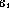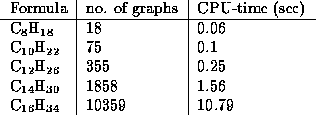Next: The use of Up: MOLGEN, a generator of... Previous: The computation of

# The use of MOLGEN in education

MOLGEN is designed both for educational purposes and for research. In chemical education it helps to illustrate the vast amount of combinatorial and geometric possibilities to connect atoms in a prescribed way to form a molecule. The student can enter a chemical formula together with valences of the occurring atoms and then he will, first of all, be shown if there exist any molecular graphs corresponding to the given brutto formula and valences at all. The next information he gets is, how many such molecular graphs there are (if there are any, and if the computer can manage the problem in question in reasonable time). For example, if he enters C, prescribes valence 3 for every carbon atom (since in fact CHis meant), together with 12 fivemembered rings of carbon atoms as macro-atoms, the generator yields 7848 simple graphs consisting of 12 points (=macro-atoms) of valence 5. In the next step of expanding the macro-atoms MOLGEN starts generation (since the total number of atoms is less than 100), but the generated number of molecular graphs will be more or less infinite, even if there are 10 nonoverlapping benzene rings prescribed as macroatoms. More moderate questions show that there are altogether 217 molecular graphs for CHthat there are exactly 22 dioxines, i.e. molecular graphs with the chemical formula COHCland the prescribed skeleton like in fig., and so on.Figure 2: Skeleton of dioxine

Some of these isomers are shown in many textbooks, too.

Further numbers and CPU times (with respect to a 486 DX2/66 running OS/2) are gathered in the following table:These numbers of isomers agree with the numbers obtained by ESESOC (cf. ), but the present generator seems to be more efficient. In general the numbers of isomers grow very rapidly, as shown in table 2.Next: The use of Up: MOLGEN, a generator of ... Previous: The computation of

Send questions to: molgen@btm2x2.mat.uni-bayreuth.de